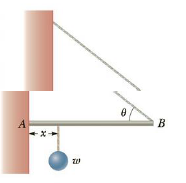Chapter 8, Problem 36P

Chapter
Section
Textbook Problem

Q One end of a uniform 4.0-m-long rod of weight w is supported by a cable at an angle of θ = 37° with the rod. The other end rests against a wall, where it is held by friction. (See Fig. P8.36.) The coefficient of static friction between the wall and the rod is μs = 0.50. Determine the minimum distance x from point A at which an additional weight w (the same as the weight of rod) can be hung without causing the rod to slip at point A.Figure P8.36

To determine

The minimum distance from a point A at which an additional weight w can be hung without causing the rod to slip at point A.

Explanation

Given info: The coefficient of static friction is 0.50 ,angle of the cable with the rod is 37° and the force of friction is 0.40T .

The frictional force at point A is f=(fs)max=μsn but the sum of horizontal forces on the rod at equilibrium condition is Fx=0=nTcosθ. By using these two expressions, the force of friction is calculated. At equilibrium condition, the vertical components of forces on the rod is defined as Fy=0=f+Tsinθ2w. The sum of torques about the point A is τ=0=xminw(l/2)w+l(Tsinθ)=0.

The formula for the force of friction is,

f=μsTcosθ

• μs is coefficient of static friction.
• T is force of tension in the cable.
• θ is the angle of the cable with the rod.

Substitute 0.50 for μs and 37° for θ to find f.

f=(0.50)(cos37°)T=0.40T

Thus, the force of friction the surfaces of the wall and the end of the rod is 0.40T

Still sussing out bartleby?

Check out a sample textbook solution.

See a sample solution

The Solution to Your Study Problems

Bartleby provides explanations to thousands of textbook problems written by our experts, many with advanced degrees!

Get Started

Find more solutions based on key concepts